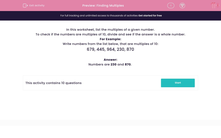# Finding Multiples

In this worksheet, students work through a number problem to find the multiples.Key stage:  KS 2

Curriculum topic:   Maths and Numerical Reasoning

Curriculum subtopic:   Factors and Multiples

Difficulty level:#### Worksheet Overview

In this worksheet, list the multiples of a given number.

To check if the numbers are multiples of 10, divide and see if the answer is a whole number.

For Example:

Write numbers from the list below, that are multiples of 10:

679, 445, 964, 230, 870